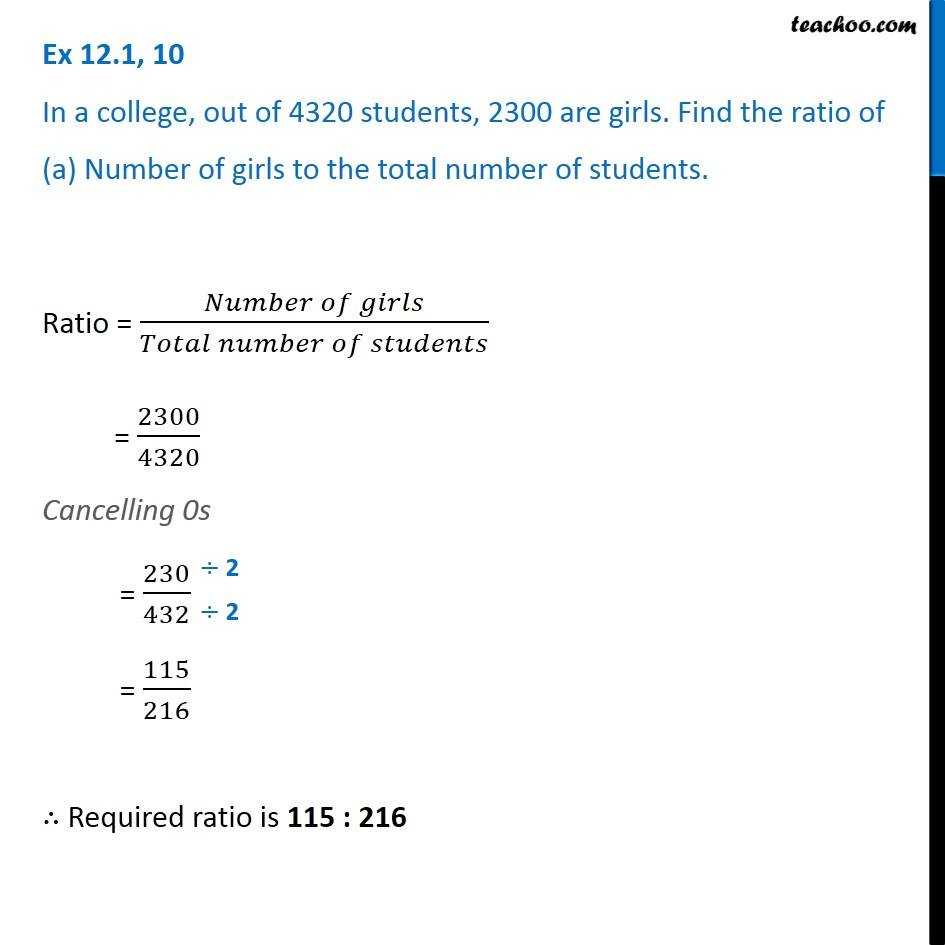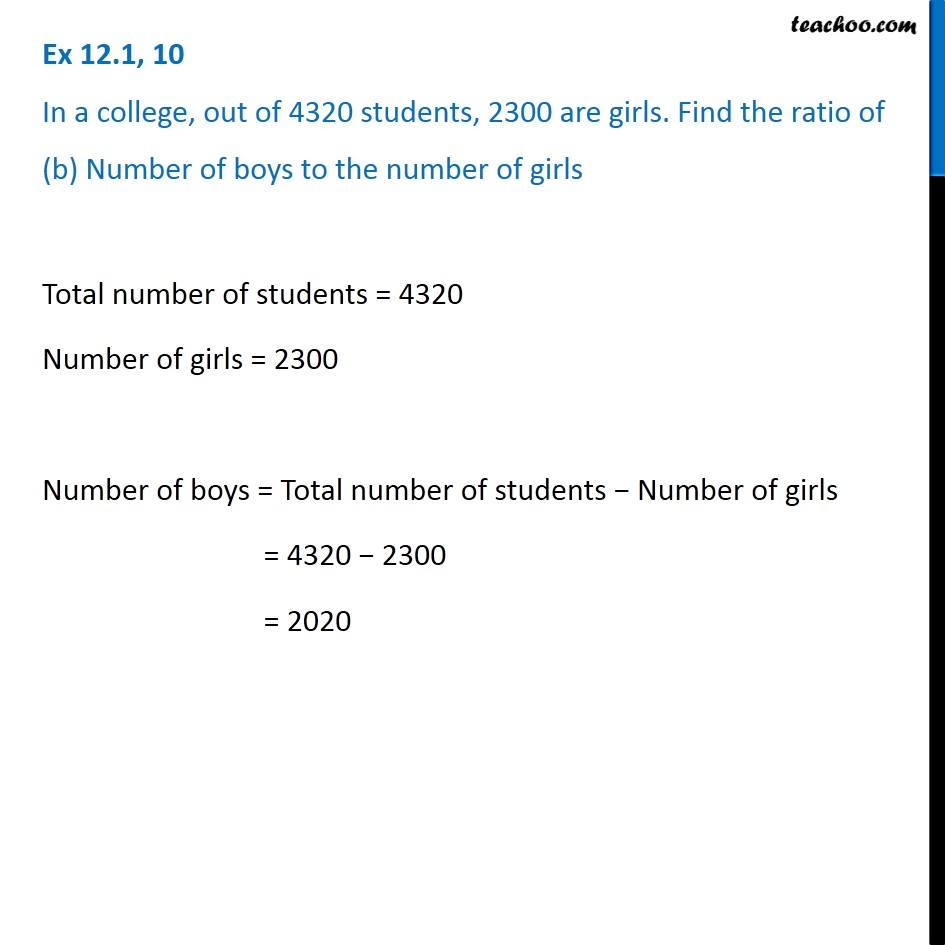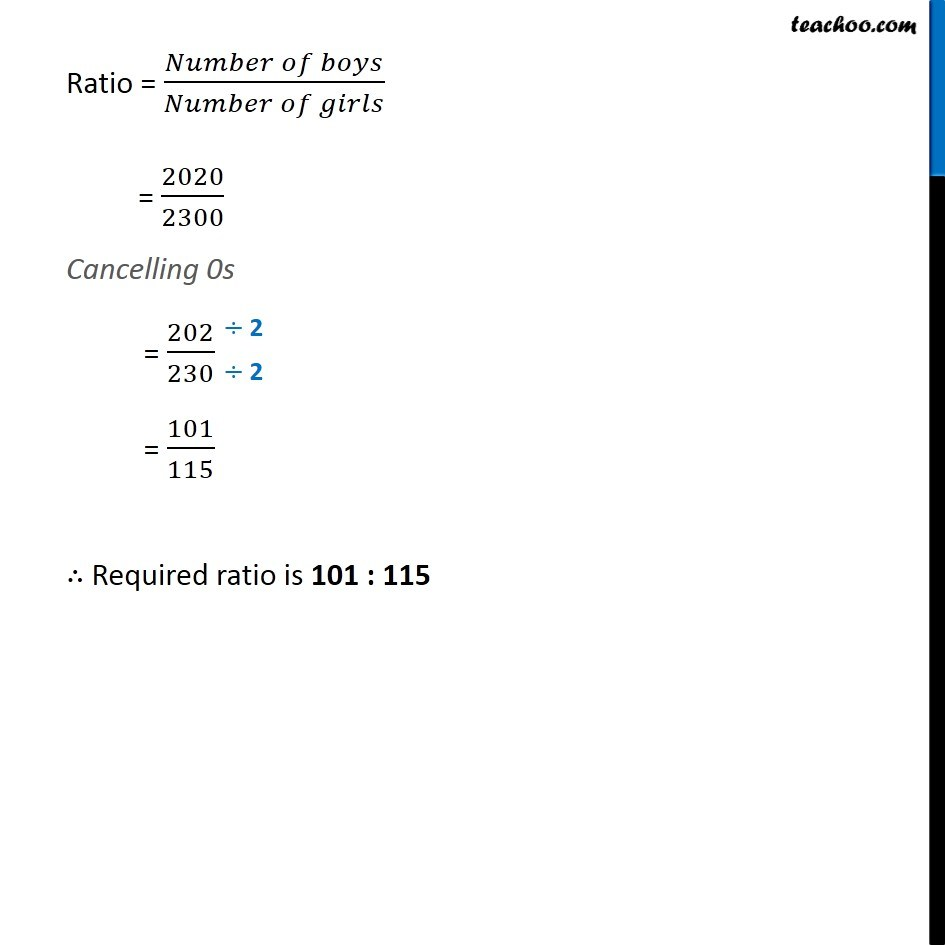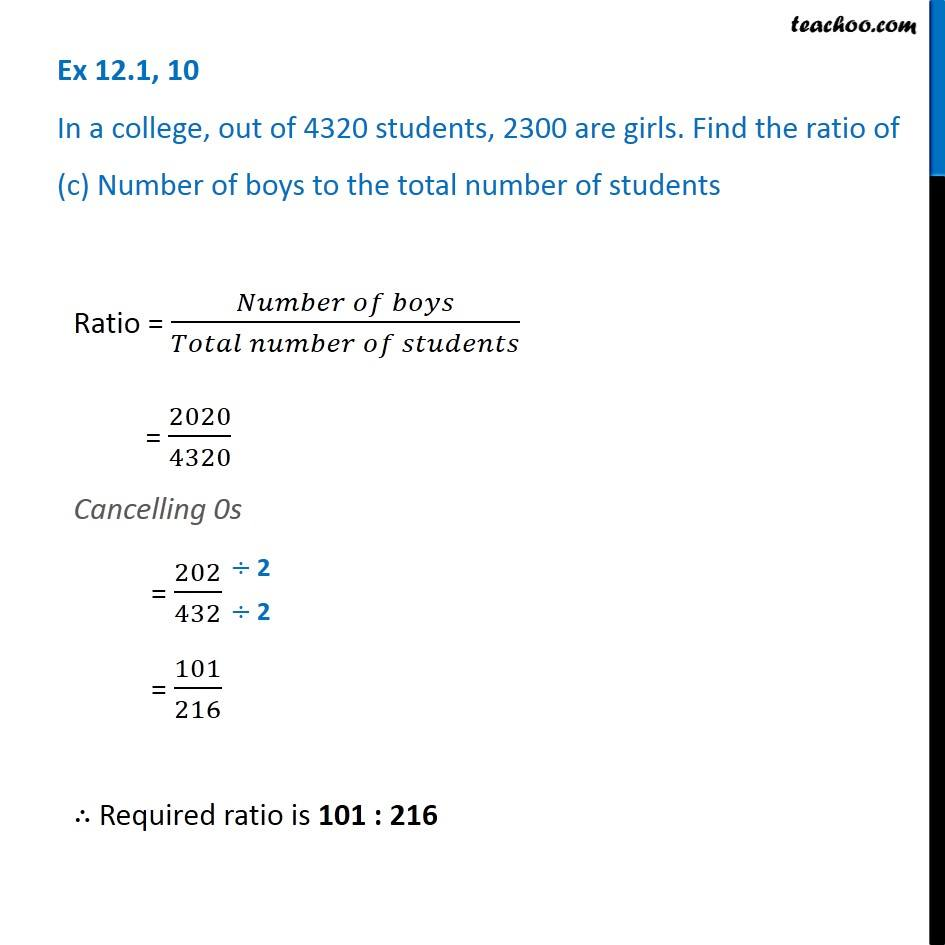Ratios

Chapter 12 Class 6 Ratio and Proportion
Concept wiseLearn in your speed, with individual attention - Teachoo Maths 1-on-1 Class

### Transcript

Ex 12.1, 10 In a college, out of 4320 students, 2300 are girls. Find the ratio of (a) Number of girls to the total number of students. Ratio = (𝑁𝑢𝑚𝑏𝑒𝑟 𝑜𝑓 𝑔𝑖𝑟𝑙𝑠)/(𝑇𝑜𝑡𝑎𝑙 𝑛𝑢𝑚𝑏𝑒𝑟 𝑜𝑓 𝑠𝑡𝑢𝑑𝑒𝑛𝑡𝑠) = 2300/4320 Cancelling 0s = 230/432 = 115/216 ∴ Required ratio is 115 : 216 Ex 12.1, 10 In a college, out of 4320 students, 2300 are girls. Find the ratio of (b) Number of boys to the number of girls Total number of students = 4320 Number of girls = 2300 Number of boys = Total number of students − Number of girls = 4320 − 2300 = 2020 Ratio = (𝑁𝑢𝑚𝑏𝑒𝑟 𝑜𝑓 𝑏𝑜𝑦𝑠)/(𝑁𝑢𝑚𝑏𝑒𝑟 𝑜𝑓 𝑔𝑖𝑟𝑙𝑠) = 2020/2300 Cancelling 0s = 202/230 = 101/115 ∴ Required ratio is 101 : 115 Ex 12.1, 10 In a college, out of 4320 students, 2300 are girls. Find the ratio of (c) Number of boys to the total number of students Ratio = (𝑁𝑢𝑚𝑏𝑒𝑟 𝑜𝑓 𝑏𝑜𝑦𝑠)/(𝑇𝑜𝑡𝑎𝑙 𝑛𝑢𝑚𝑏𝑒𝑟 𝑜𝑓 𝑠𝑡𝑢𝑑𝑒𝑛𝑡𝑠) = 2020/4320 Cancelling 0s = 202/432 = 101/216 ∴ Required ratio is 101 : 216